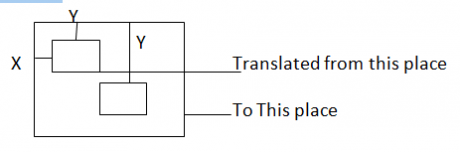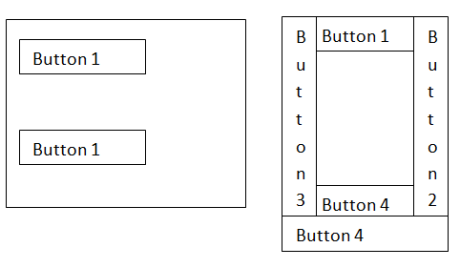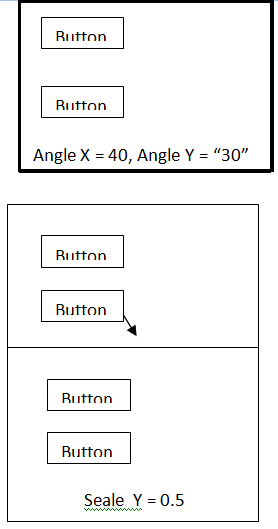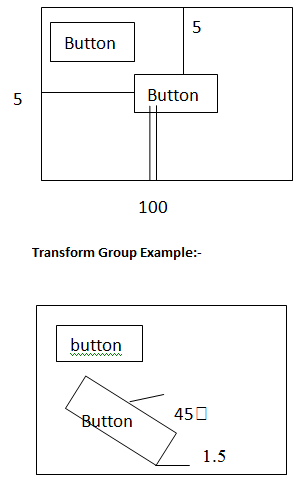# SilverLight Transforms

Blog Author

Tekslate

Published Date

20th September, 2018

Ratings

Views

812

The transformation is used to change one coordinate space to another co ordinate space, the transformation Microsoft designed using 3x3 matrix.

Transforms are used to apply the required effects to the 2D and 3D objects. Effects are like rotating, tilling the object, stretching as well as shrinking etc. using transforms we can give different effects to the controls

The transformation we can classify in to five types

1. Rotate transform
2. Scale transform
3. Skew transform
4. Translate transform
5. Matrix transform

## Rotate Transform

using this transformation we can rotate an object using angle property.

## Scale Transform

using this transformation we can change x-co ordinate space and y-co ordinate space in the silverlight control, for changing co ordinate spaces we have to work with scale X, scaley properties.

## Skew Transform

using this transformation we can skew an object co ordinate spaces using angles x, angle y properties.

## Translate Transform

using this transformation we can set control X location and Y location for changing the locations the Microsoft given X and Y properties.

## Example on Transformations

Open new silver light application

Go to XAML mode

In the canvas tag

<Canvas>

<Image X: name = “11” height = “100” width = “100” source = “ d:/face .jpg” canvas. Left = “100”>

<Image. Render transform>

<Rotate Transform Angle = “50”> </rotate Transform>

</Image. Render transform>

</Image>

<Image X:Name = “i2” Height = “100” width = “100” source = “d:/face.jpg” canvas. Left = “200”>

<image. Render transform >

<scale transform scale x = “5” scale y = “1” > </scale transform>

</image. Render transform>

</Image>

<image X:name = ”i3” height = “100” width = “100” source = “d : / face .jpg” canvas. Top = “170”>

<image. Render transform>

]<skew transform anglex = “10” angle Y = “20”> </skew transform>

</Image. Rendertansform>

</image>

</image x:Name = “i4” height = “100” width = “100” source = “d: /face .jap canvas. Left = “250” canvas. Top = “200” >

<image. Render transform>

<Translate transform X = “10” Y = “10”> </Translate Transform>

</image. Render transform>

</Image>

</canvas>

Image. Render Transform: This tag is used to create workspace for defining transformations.

• Matrix Transform:

This transformation used to implement customized or programmer defined transformation, matrix transformation is combination of first four transformations.

This transformation the Microsoft designed using 3 x 3 matrix, depending on the requirement we can change any coordinate value

### Matrix transform properties

M11: using this property we can set first row, first column values from the 3x3 matrix

M12 : using this property we can change first row, second colum values.

M21: using this property we can change second row and first column values

M22: using this property we can change second row and second column values.

Offset X:  using this property we can change third row and first column values

Offset Y:  using this property we can change third row and second column values

## Example on Matrix Transformation

Open new silver light application

Go to XAML Mode

In the canvas tag

<canvas>

<list box x: name = “l1” Height = “250” canvas.left = “50” canvas. Top = “100”>

<list box item>

<stack panel orientation = “horizontal’>

<text block text = “one”> </text block>

<Image source = “d:/one .jpg” Height = “40” > </imge>

</stack panel>

</list box item>

<listbox item>

<stack panel orientation = “horizontal”>

<Text block text = “Two”>

</text block>

<Image source = “d:/two.jpag” Height = “40”> </image>

</stack panel>

</list box item>

<list box item>

<stack panel orientation = “horizontal”>

<text block Text = “Three” > </Textblock>

<image source = “d:/three.jpg” height = “40”> </image>

</stack panel>

</list Box Item>

<list box item>

<stack panel orientation = “Horizontal”>

<Text block text = “three” > </Text block>

<Image source = “d:/three.jpg” height = “40” > </image>

</stack panel>

</list box item>

<listbox. Render transform>

<matrix transform>

<matrix transform. Matrix>

<matrix M11 = “1” M12 = “-.51” Mel = “.51”

M22 = “3” offset x = “10” offset y = “10”>

</matrix>

</matrix transform . matrix>

</matrix transform>

</list box. Render transform>

</list box>

</canvas>

### List Box

This tag is used to create list box control, the list box control maintains list of items, depending on the selected item we can give the information.

### List box item

This tag is used to add items in the list box control, each item we can give using silverlight controls, by default we can take one item, when we are taking multiple control features in the single item we have to work with stack panel layout.

Matrix Transform. Matrix :  This tag gives workspace for defining matrix transformation.

### Example on double animation with transformation:

Open new silverlight application

Go to XAML Mode

In the canvas tag

<canvas>

<Ellipse X:name = “e1” Height = “100” width = “150” stroke = “red” stroke thickness = “10” canvas. Left = “50” canvas. Top = “50” mouse Enter = “e1_mouseenter”>

</Ellipse. File>

<Ellipse. Render transform>

<skew transform angle x = “1” angley = “1” > </skew transfer>

</ellipse. Render transform>

<ellipse. Resource>

<storyboard x:name = “s1”>

<double animation storyboard. Target name = “e1” storyboard. Target property = “(UIElement. Render transform). Am from = “1” To = “70” auto reverse = “True” duration = “0:0:10”>

<\bouble animation >

<double animation storyboard. Target name = “e1” storyboard. Target property = “(UIElement. Render transform). And from = “1” to = “70” auto reverse = “true” duration = “0”0”10”>

</double animation>

</story board>

</ellipse. Resource>

</ellipse >

</canvas>

El_mouse enter

{

1. Begin ();

}

## Types of Transforms

• Silver light will support following types of trans
• Rotate transform
• Skew transform
• Scale transform

### Syntax to implement Transforms

<Control class name attributes> <control class name Render Transform> <Transform class name property 1= “value” property 2=”value”/>|

</control class name.render Transform> </confrol class name>

## Rotate Transform:-

This is used to rotate the object in clock wise or anti clock wise about a specified point in a two dimensional x,y coordinate system. When we use rotate transform the transformation rotates the coordinate system for a particular object about the origin point.

Every type of transform has a special class with same name of the transform.

• Angle
• Center X
• Center Y

### Angle

This property is used to set or get the required angle in which the object should be rotated. If we give the angle as positive the rotation will be in clock wise. If the angle is  negative the rotation will be in anticlockwise

### Center X & center Y

Used to mention the origin or the angle to be rotated in both X and Y  directions.

## Skew Transform

this is used to filt the object in required angle. Of X and Y coordinates. A skew Transform is useful for creating illusion of

Properties of skew Transform class

• Angle X
• Angle Y
• Center X
• Center Y

### Angle X

Used to set/get the required angle value that is to be filter form X-axis.

### Angle Y

Used to set/get a value that is to be tilted from Y axis

## Scale Transform

This transform is user to stretch or shrink an object horizontally or vertically.  This transformation is used to scale an object in the two dimensional x,y coordinate system

### Properties of scale Transform class

• Scale X
• Scale Y
• Center X <scale X=”1.5”
• Center Y

### Scale X

This property is used to set or get the value that is used to be shrink |stretch in X direction

### Scale Y

used t set or get a value that indicates to shrink |stretch in Y direction

When we implement scale transform the object current state is assumed to ‘1’ the object will be stretched and if we are giving a value of less then ‘1’ the object will be shirked

## Translation Transform

This transform is used to move the current object in two dimensional x,y coordinate system

Properties:-X Y

X:-Used to set 1 get the required value that the object should be moved in x-direction

Y:-Used to set 1 get a value that the object should be moved in Y direction.

### Transform Group

This transform is used to apply two 0.2 more transforms at once to the object. In general using render transform we can apply only one transform at once there are no special properties for transform group, Rather it will contain other 4 transform classes as a group with in it.

From the other four transforms, we can implements two or more transforms together using this transform group class

### Example with rotate transform class

<content=”Button”>

Create a button within the silver light application page go to .xaml source and write the following code <Button content+”Button” Height=”28” width=”60” content=”Button”<button. Render transform> <Relate Transform AngleX=”45”/> </Button.Renter Transform>

### Example with skew transform.

<Grid x: name=layout Rool “Background = “while”> <button content= “Button” Height = “28” Width = “60” content= “Button1”>

<button render transform>

<Skew transform Angle x = “40” Angle Y= “30”/>

</Button. Render Transform>

</Button>### Example with scale Transform:-

<button content = “Button” Height = “28”--- <Button. Render transform> <Scale Transform scale X = “2.5” Scale Y = “1.5”/>

</Button. Render Transform> </Button>

### Example of Translation Transform

<button Content> <button.Render Transform> <Translation Transform X=”100” Y = “5”/> </Button.Render Transform> </Button>### Transform Group Example

<button content = Button <Button. Render Transform> <Transform Group>

<Rotate Transform Angle = “45”/> <Scale Trans form scale X= “1.5”/>

</TransformGroup> </button.RenderTransform> </Button